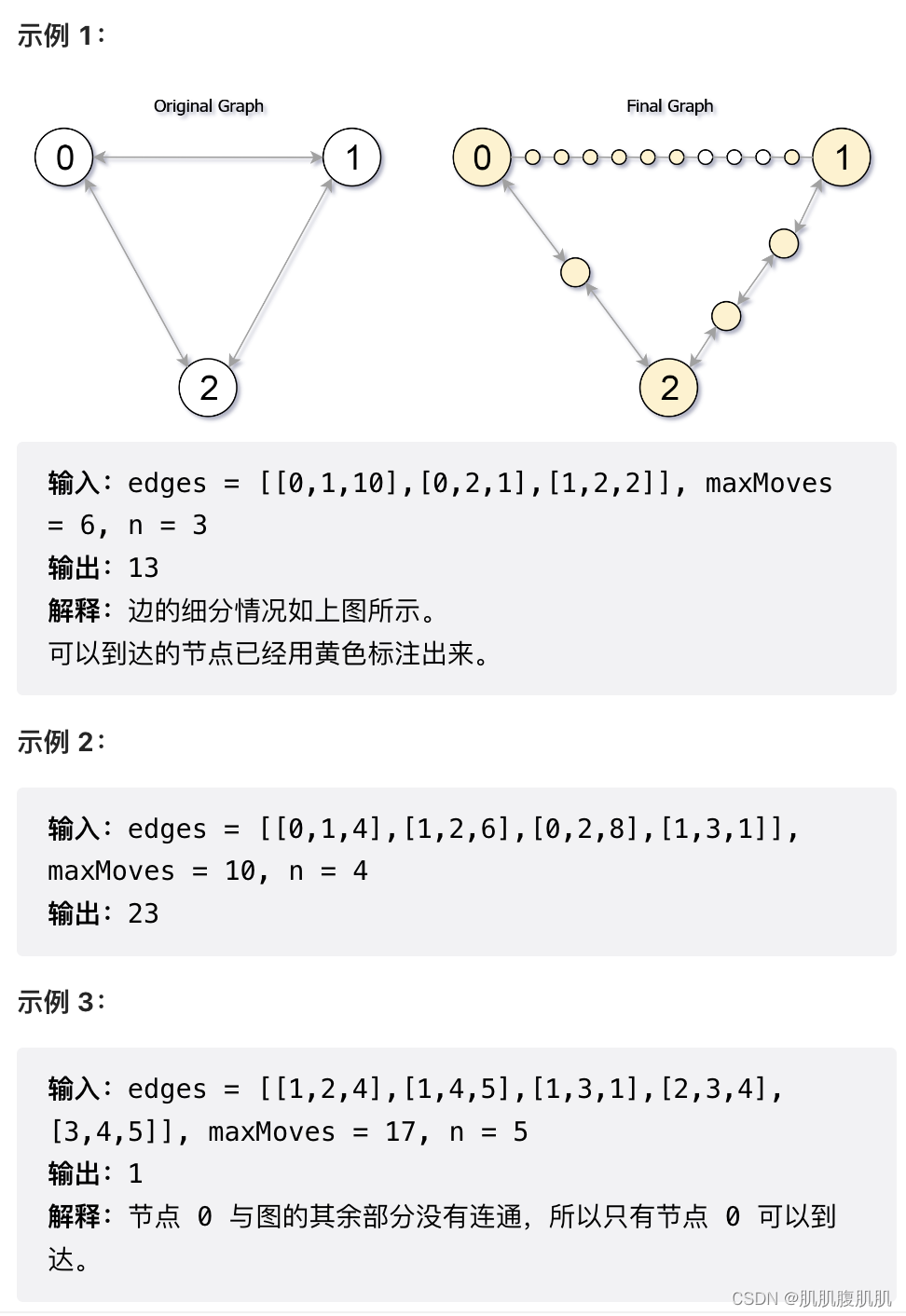# 最短路算法 - dijkstra

### 最短路算法 - dijkstra

• 1. 算法介绍
• 2. 实战
• 2.1 Reachable Nodes In Subdivided Graph
• 3 参考

# 1. 算法介绍

1. 初始化距离数组 dis 和优先级队列，其中 dis[i] 表示 s 点到当前 i 点的最短距离，优先级队列储存的是已经求出最短距离的点，按与 s 点之间的距离从小到大排序；
2. 每次取出距离 s 最近的一个点 c，遍历 c 所能到达的相邻点 n，尝试更新 s 点到 n 的距离（如果 dis[c] + c 到 n 之间的距离 < dis[n], 就更新 dis[n] = dis[c] + c 到 n 之间的距离），并将 n 加入优先级队列；
3. 重复第二步，直至队列为空。

# 2. 实战

## 2.1 Reachable Nodes In Subdivided Graph

Leetcode: 882. Reachable Nodes In Subdivided Graph``````class Solution {
public int reachableNodes(int[][] edges, int maxMoves, int n) {
List<int[]>[] g = new ArrayList[n];
Arrays.setAll(g, e -> new ArrayList<>());
for (int[] pair : edges) {
int from = pair, to = pair, edge = pair + 1;
}

PriorityQueue<int[]> pq = new PriorityQueue<>((e1, e2) -> e1 - e2);
pq.offer(new int[]{0, 0});
int[] dis = new int[n];
Arrays.fill(dis, maxMoves + 1);
dis = 0;
boolean[] visited = new boolean[n];
while (!pq.isEmpty()) {
int curr[] = pq.poll();
int currNode = curr;
if (visited[currNode]) continue;
visited[currNode] = true;
for (int[] next : g[currNode]) {
int nextNode = next, nextDis = next;
if (!visited[nextNode] && dis[currNode] + nextDis < dis[nextNode]) {
dis[nextNode] = dis[currNode] + nextDis;
pq.offer(new int[]{nextNode, dis[nextNode]});
}
}
}
int ans = 0;

for (int i = 0; i < n; i++) {
if (dis[i] <= maxMoves) {
ans++;
}
}

for (int[] pair : edges) {
ans += Math.min(pair, Math.max(0, maxMoves - dis[pair]) + Math.max(0, maxMoves - dis[pair]));
}

return ans;

}
}
``````

# 3 参考

• 【LeetCode】882. Reachable Nodes In Subdivided Graph
• 又快又准做对考研真题，从考试的角度出发【Dijkstra】【单源最短路径】试利用Dijkstra算法求下图中从顶点a到其他各顶点间的最短路径，写出执行算法过程中